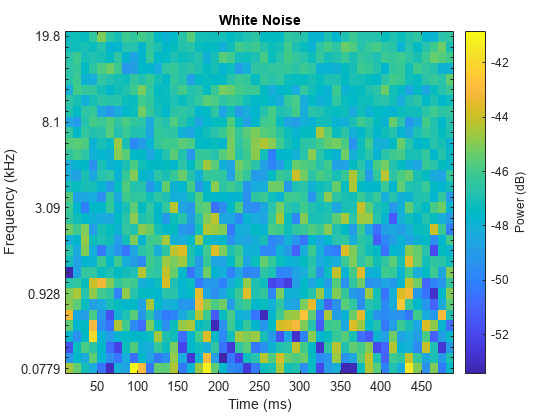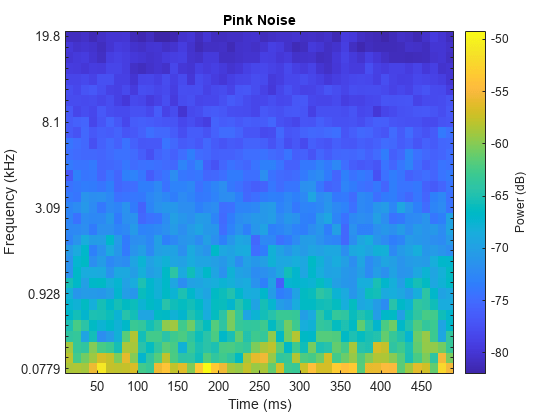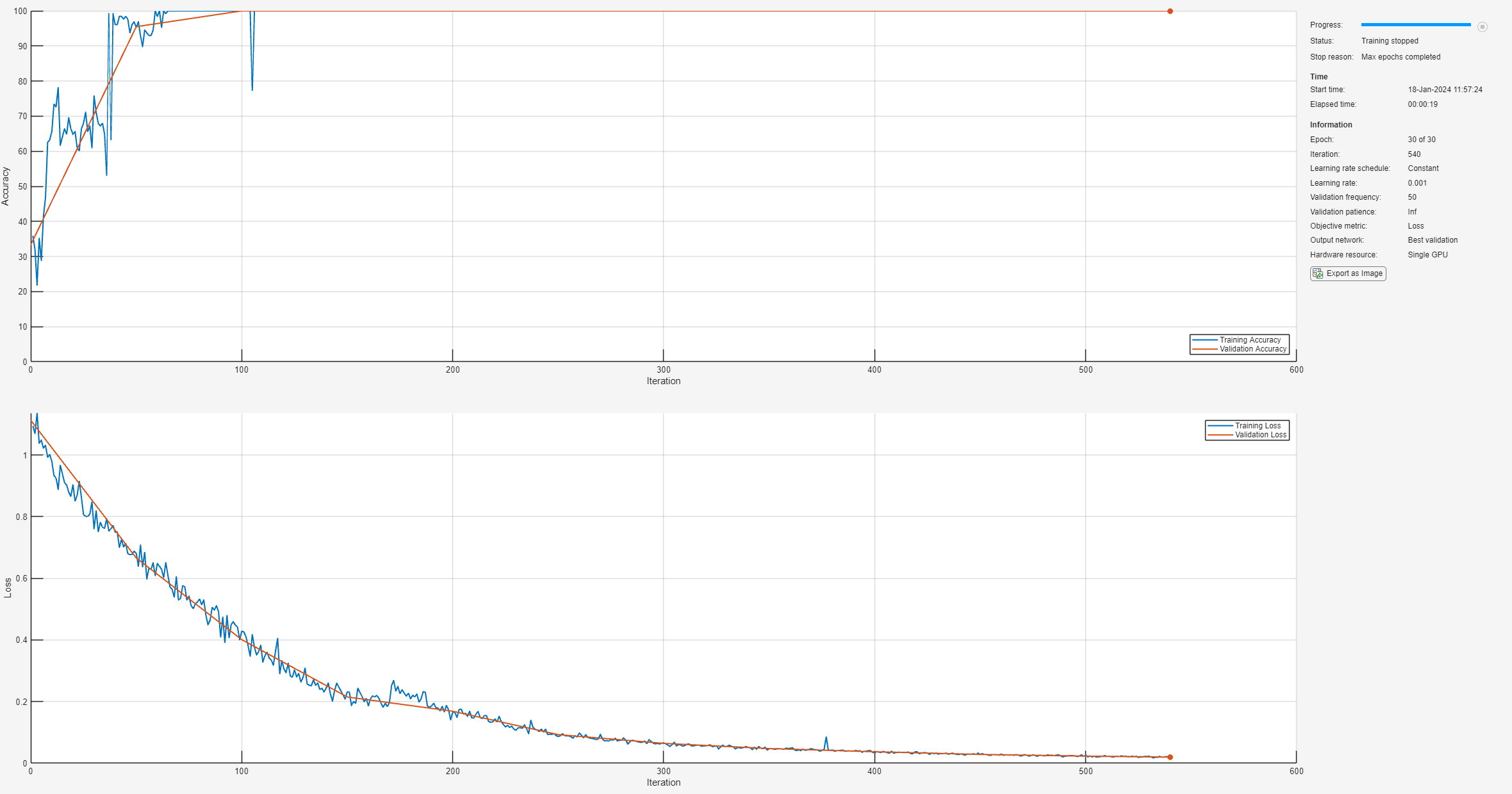# Classify Sound Using Deep Learning

This example shows how to classify a sound by using deep learning processes.

### Create a Data Set

Generate 1000 white noise signals, 1000 brown noise signals, and 1000 pink noise signals. Each signal represents a duration of 0.5 seconds, assuming a 44.1 kHz sample rate.

```fs = 44.1e3; duration = 0.5; N = duration*fs; wNoise = 2*rand([N,1000]) - 1; wLabels = repelem(categorical("white"),1000,1); bNoise = filter(1,[1,-0.999],wNoise); bNoise = bNoise./max(abs(bNoise),[],'all'); bLabels = repelem(categorical("brown"),1000,1); pNoise = pinknoise([N,1000]); pLabels = repelem(categorical("pink"),1000,1);```

### Explore the Data Set

Listen to a white noise signal and visualize it using the `melSpectrogram` `function`.

```sound(wNoise(:,1),fs) melSpectrogram(wNoise(:,1),fs) title('White Noise')```Inspect a brown noise signal.

```sound(bNoise(:,1),fs) melSpectrogram(bNoise(:,1),fs) title('Brown Noise')```Inspect a pink noise signal.

```sound(pNoise(:,1),fs) melSpectrogram(pNoise(:,1),fs) title('Pink Noise')```### Separate the Data Set into Train and Validation Sets

Create a training set that consists of 800 of the white noise signals, 800 of the brown noise signals, and 800 of the pink noise signals.

```audioTrain = [wNoise(:,1:800),bNoise(:,1:800),pNoise(:,1:800)]; labelsTrain = [wLabels(1:800);bLabels(1:800);pLabels(1:800)];```

Create a validation set using the remaining 200 white noise signals, 200 brown noise signals, and 200 pink noise signals.

```audioValidation = [wNoise(:,801:end),bNoise(:,801:end),pNoise(:,801:end)]; labelsValidation = [wLabels(801:end);bLabels(801:end);pLabels(801:end)];```

### Extract Features

Audio data is highly dimensional and typically contains redundant information. You can reduce the dimensionality by first extracting features and then training your model using the extracted features. Create an `audioFeatureExtractor` object to extract the centroid and slope of the mel spectrum over time.

```aFE = audioFeatureExtractor("SampleRate",fs, ... "SpectralDescriptorInput","melSpectrum", ... "spectralCentroid",true, ... "spectralSlope",true);```

Call extract to extract the features from the audio training data.

```featuresTrain = extract(aFE,audioTrain); [numHopsPerSequence,numFeatures,numSignals] = size(featuresTrain)```
```numHopsPerSequence = 42 ```
```numFeatures = 2 ```
```numSignals = 2400 ```

In the next step, you will treat the extracted features as sequences and use a `sequenceInputLayer` as the first layer of your deep learning model. When you use `sequenceInputLayer` as the first layer in a network, `trainNetwork` expects the training and validation data to be formatted in cell arrays of sequences, where each sequence consists of feature vectors over time. `sequenceInputLayer` requires the time dimension to be along the second dimension.

```featuresTrain = permute(featuresTrain,[2,1,3]); featuresTrain = squeeze(num2cell(featuresTrain,[1,2])); numSignals = numel(featuresTrain)```
```numSignals = 2400 ```
`[numFeatures,numHopsPerSequence] = size(featuresTrain{1})`
```numFeatures = 2 ```
```numHopsPerSequence = 42 ```

Extract the validation features.

```featuresValidation = extract(aFE,audioValidation); featuresValidation = permute(featuresValidation,[2,1,3]); featuresValidation = squeeze(num2cell(featuresValidation,[1,2]));```

### Define and Train the Network

Define the network architecture. See List of Deep Learning Layers (Deep Learning Toolbox) for more information.

```layers = [ ... sequenceInputLayer(numFeatures) lstmLayer(50,"OutputMode","last") fullyConnectedLayer(numel(unique(labelsTrain))) softmaxLayer classificationLayer];```

To define the training options, use `trainingOptions`.

```options = trainingOptions("adam", ... "Shuffle","every-epoch", ... "ValidationData",{featuresValidation,labelsValidation}, ... "Plots","training-progress", ... "Verbose",false);```

To train the network, use `trainNetwork`.

`net = trainNetwork(featuresTrain,labelsTrain,layers,options);`### Test the Network

Use the trained network to classify new white noise, brown noise, and pink noise signals.

```wNoiseTest = 2*rand([N,1]) - 1; classify(net,extract(aFE,wNoiseTest)')```
```ans = categorical white ```
```bNoiseTest = filter(1,[1,-0.999],wNoiseTest); bNoiseTest= bNoiseTest./max(abs(bNoiseTest),[],'all'); classify(net,extract(aFE,bNoiseTest)')```
```ans = categorical brown ```
```pNoiseTest = pinknoise(N); classify(net,extract(aFE,pNoiseTest)')```
```ans = categorical pink ```